Basic Mathematics You Should Mastered

0
0
01. 云栖社区>
2. 博客>
3. 正文

Basic Mathematics You Should Mastered

wangxiaocvpr 2017-08-17 21:24:00 浏览505

Basic Mathematics You Should Mastered

2017-08-17  21:22:40

1. Statistical distance

In statisticsprobability theory, and information theory, a statistical distance quantifies the distance between two statistical objects, which can be two random variables, or two probability distributions or samples, or the distance can be between an individual sample point and a population or a wider sample of points.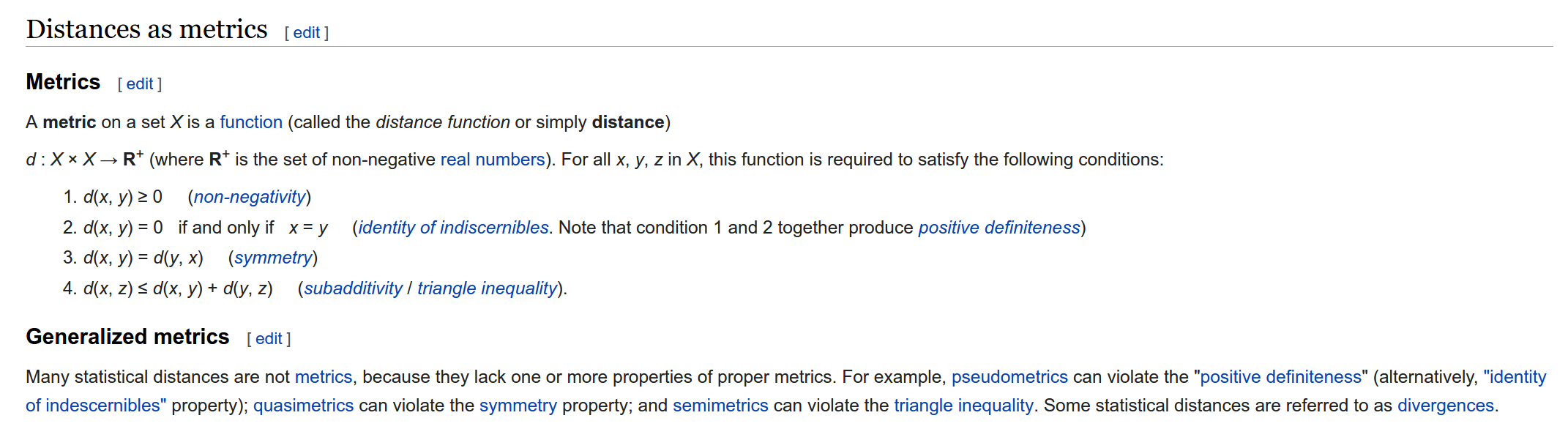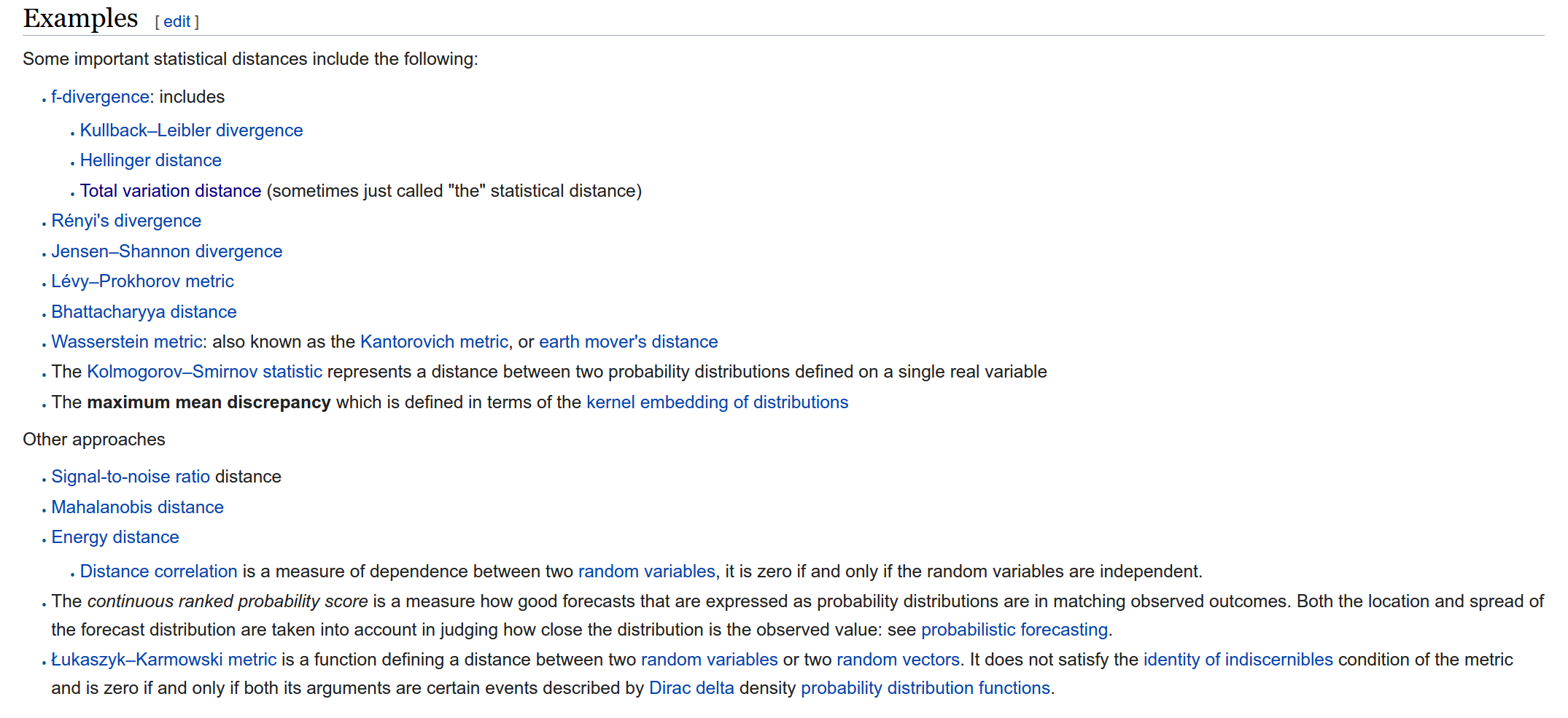2. Pinsker's inequality

In information theoryPinsker's inequality, named after its inventor Mark Semenovich Pinsker, is an inequality that bounds the total variation distance (or statistical distance) in terms of theKullback–Leibler divergence. The inequality is tight up to constant factors.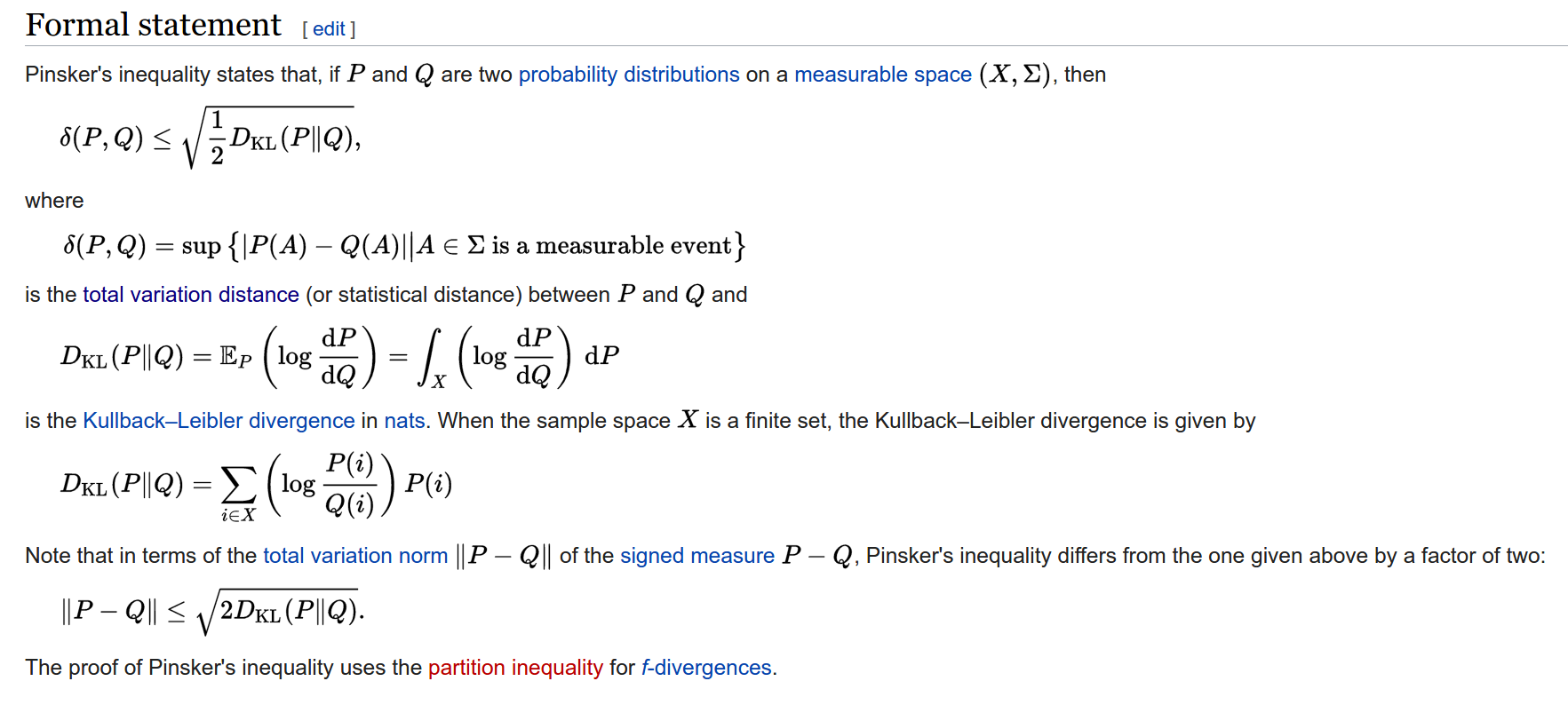3. Total variation distance of probability measures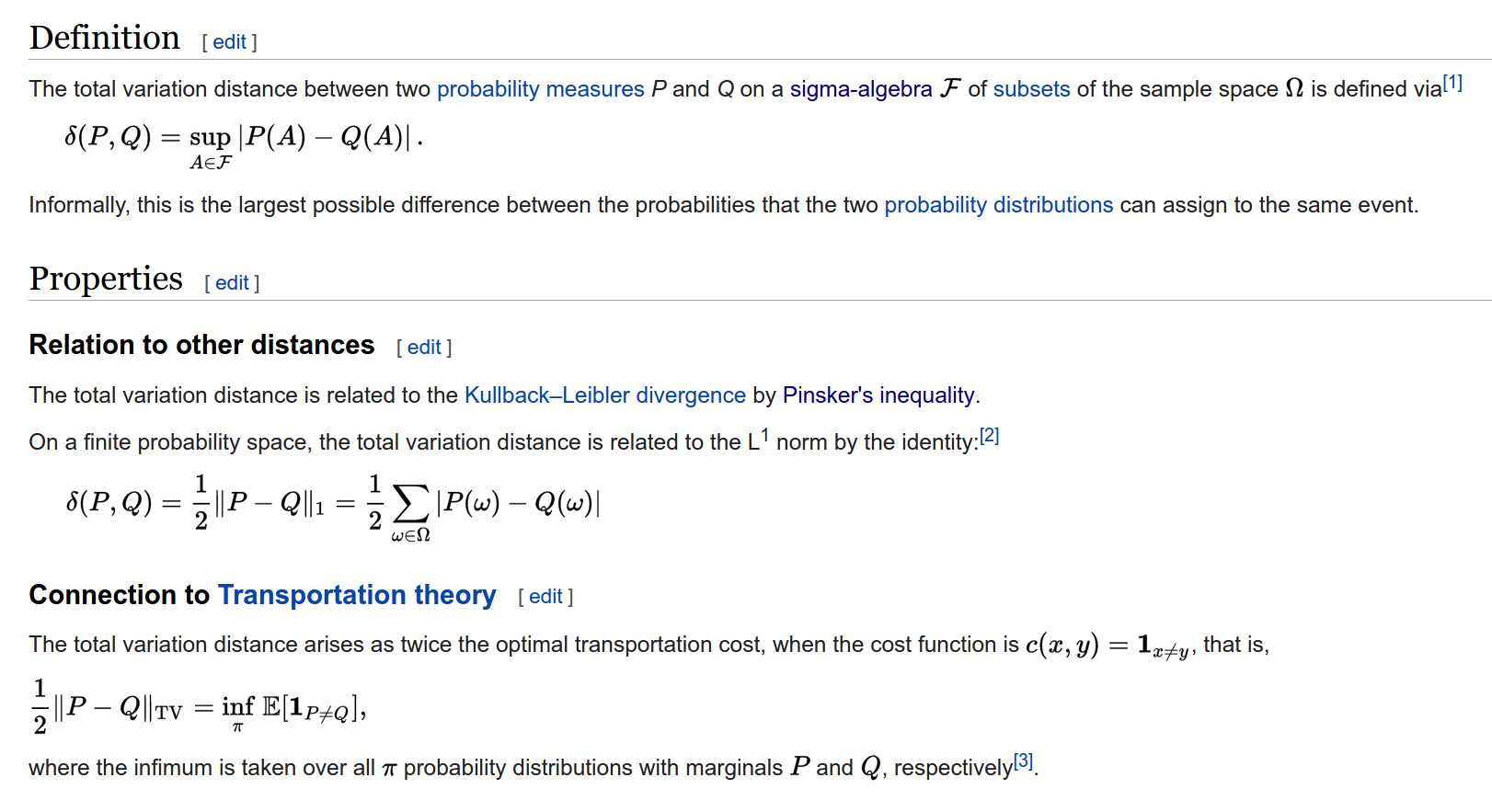4. Total variation distance of probability measures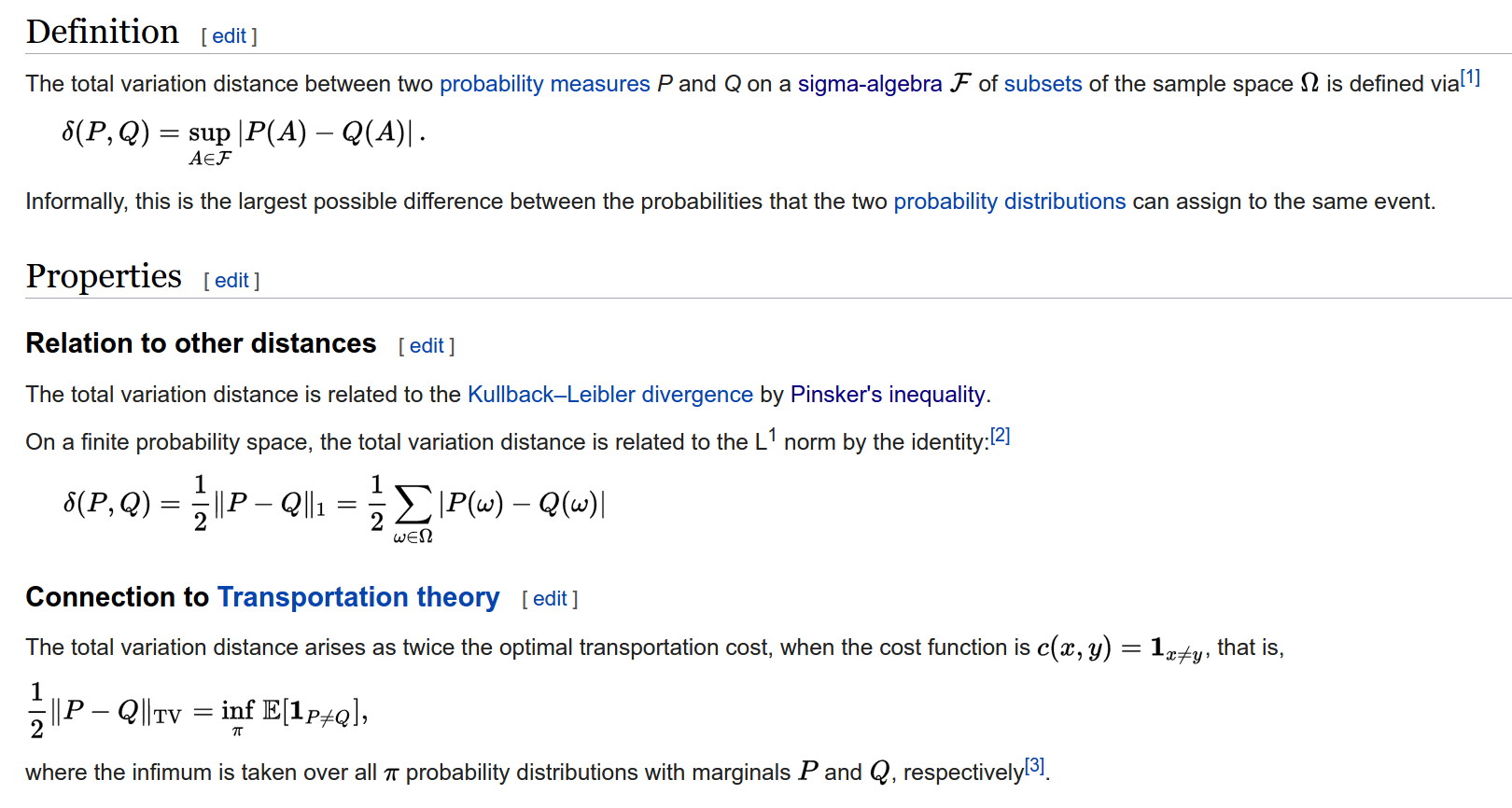5. σ-代数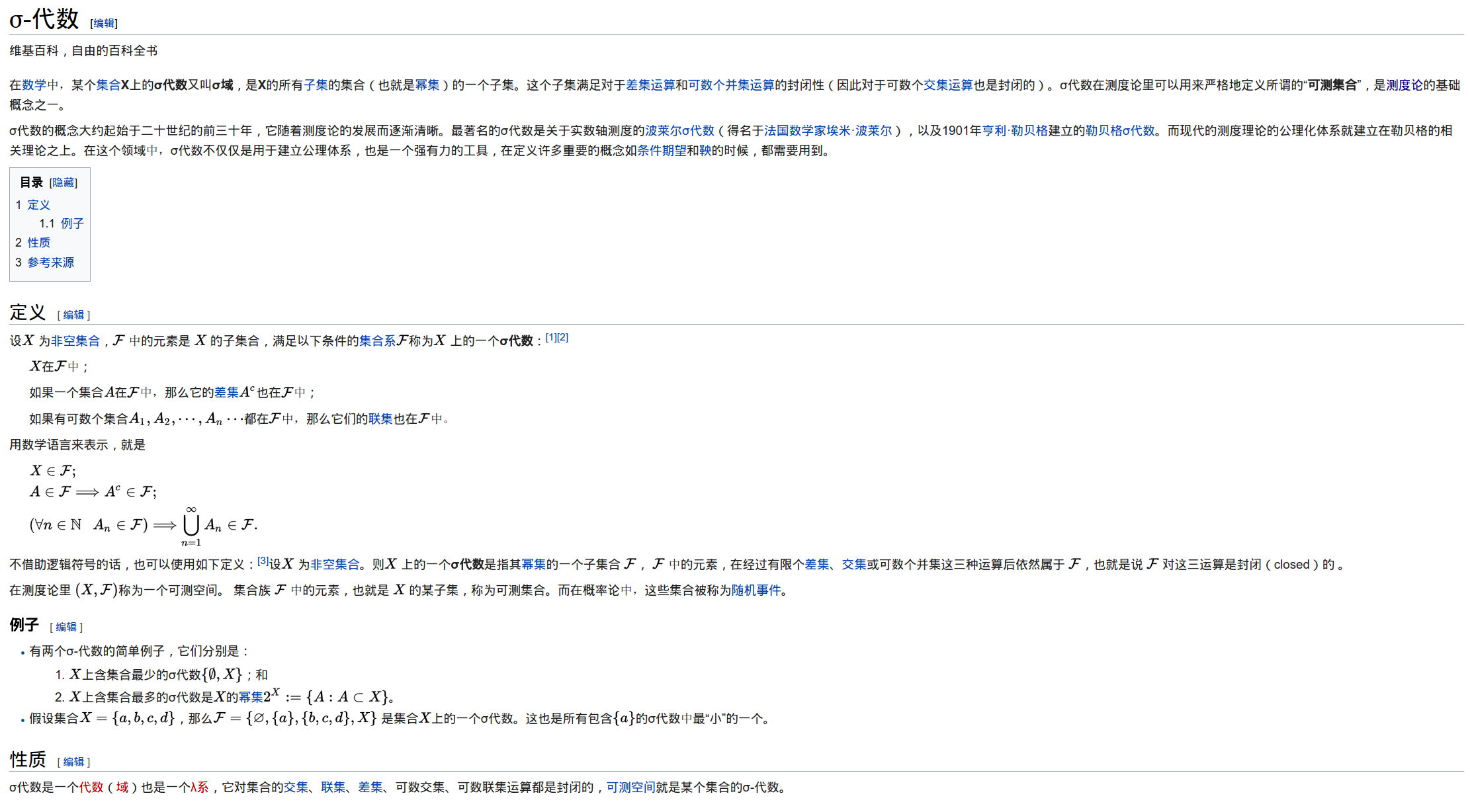6. The definition of TV: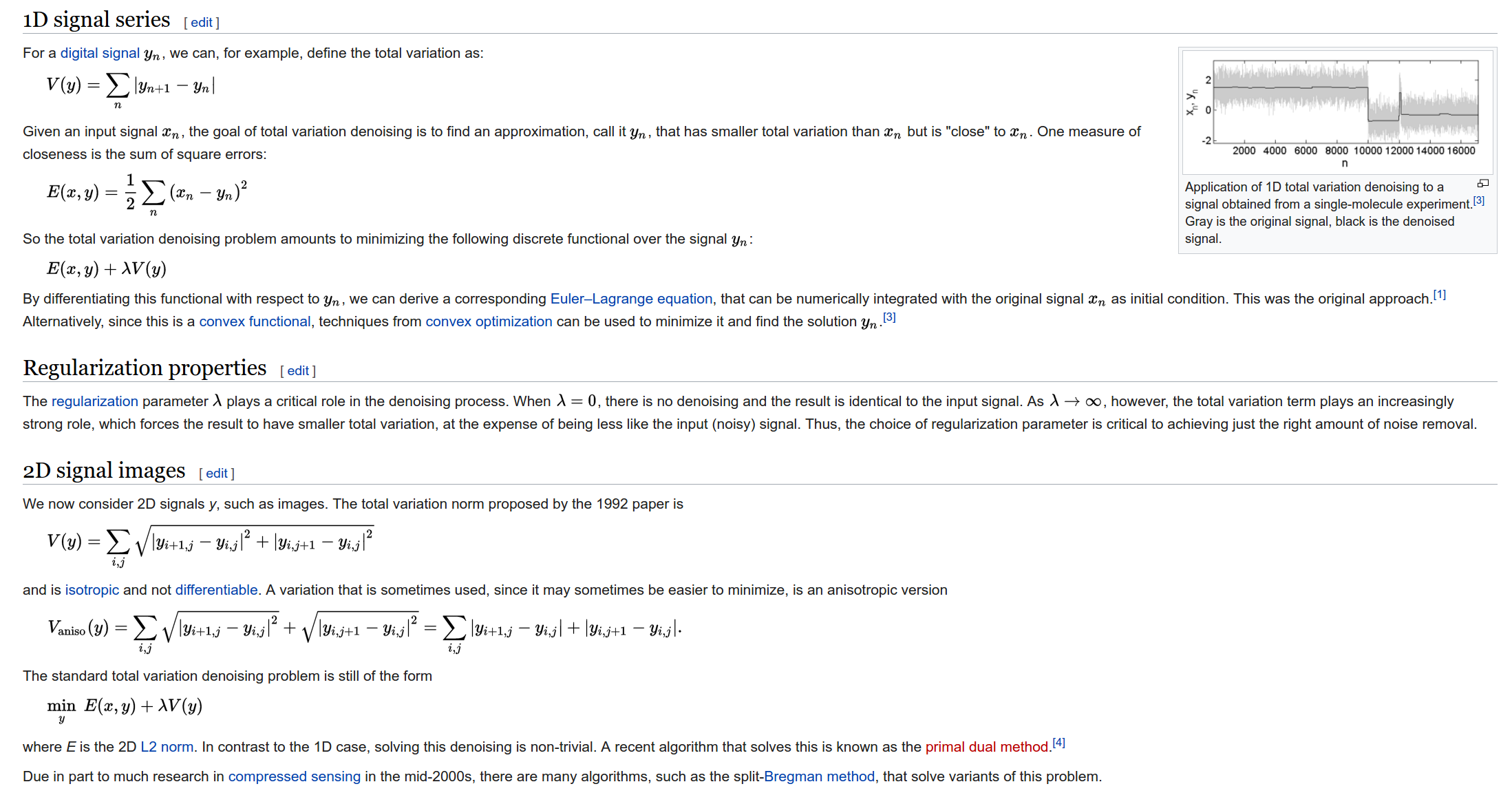wangxiaocvpr
+ 关注